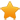Switch Editions?
CancelChannel: CodeSection,代码区,网络安全 - CodeSec
Viewing all articles

# Having bit of party with Material Colour Palette

0
0

Continuing on with my “slight?” obsession with colours… I love colours in “ Material Colour Palette ”. There various website that will lets you grab the colours by clicking, such as this one , but I just wanted to have little handy cheet sheet for myself, so I’ve decided I’ll do that using R & my favourite ggplot2 .

Getting colours out of image using package “imager”

After quick search, I came across image with all the material colour , so first things I’ve tried is to get colours out of image using imager .

## Load up packages we'll use library(tidyverse) library(imager) library(patchwork) im <- load.image("https://www.materialui.co/img/material-colors-thumb.png") #plot(im) ## Convert Image to Data Frame with HSV value im_hsv <-im %>% RGBtoHSV() %>% as.data.frame(wide="c") %>% rename(h=c.1, s=c.2, v=c.3) ## Convert Image to Data Frame with RGB value im_rgb <- im %>% as.data.frame(wide="c") %>% rename(red=c.1,green=c.2,blue=c.3) %>% mutate(hexvalue = rgb(red,green,blue)) ## you can create hexvalue using red, green blue value! ## Might as well conver to grayscale, and get luminance. ## I;ll use luminance value to decide if I'll put black text vs white text later. im_grayscale <- im %>% grayscale() %>% as.data.frame() %>% rename(luminance=value) ## I want to grab pixel from about middle of each cell mat_color <- im_rgb %>% filter(x %in% as.integer(round(seq(1,19)*(400/19))-10) & y %in% as.integer(round(seq(1,11)*(225/11))-10) & y>10) %>% left_join(im_hsv) %>% left_join(im_grayscale) %>% mutate_at(c("x","y"), dense_rank) %>% arrange(x,y) col_group <- c("red","pink","purple","deep purple","indigo","blue","light blue","cyan","teal","green","light green","lime","yellow","amber","orange","deep orange","brown","grey","blue grey") ## Adding extra info to the table mat_color <- mat_color %>% mutate(hue_group=factor(x, labels=col_group, ordered=T), shade = factor(y, labels=c(50,seq(100,900, by=100)), ordered=T)) ## I could also save this as csv file too... :) #mat_color %>% write_csv("MaterialColour.csv")

Creating Material Colour Palette Cheat Sheet

Now that I have the colours out of image in data frame, I can do fun stuff, plotting!! I should print this with colour printer, and have it as one of cheat sheet collection .

I’ve used luminance value of each colour to decide if I should place black text or white text over the colour. (I couldn’t figure out if there’s good rules to follow, but seems like luminance does the trick?!)

mat_color %>% ggplot(aes(x=hue_group,y=shade)) + ## I could also use x=x,y=y geom_tile(aes(fill=hexvalue),color="white", size=0.1) + ## i want to have very fine white line around each tiles. scale_fill_identity(guide="none") + theme_void(base_family="Roboto Condensed") + ## print out color hue name and shade geom_text(aes(label=paste0(hue_group,"-",shade), color=ifelse(luminance>0.5,"#000000","#ffffff")), ## about 48% opacity family="Roboto Condensed", size=3, vjust=-2, lineheight=0.8, alpha=0.48) + ## print out hesvalue - I'll use this the most, so print it with higher transparency geom_text(aes(label=hexvalue, color=ifelse(luminance>0.5,"#000000","#ffffff")), ## about 80% opacity family="Roboto Condensed", fontface="bold",vjust=0, alpha=0.8) + ## print out RGB & HSV geom_text(aes(label=paste0("\n(",round(red*255),",",round(blue*255),",",round(green*255),")\n(", round(h),",",round(s,1),",",round(v,1),")"), color=ifelse(luminance>0.5,"#000000","#ffffff")), ## about 67% opacity family="Roboto Condensed", size=3, vjust=1, lineheight=0.8, alpha=0.67) + scale_color_identity() + labs(x="",y="",title="", caption="Material Colour Palettes Hexvalue + RGB + HSV")

## I can save as PNG file too with below line #ggsave("MaterialColorCheatSheet.png", width=16, height=9)

More Parrrty Time with Colours…

While plotting colour in rectangular is good…. I thought it’s a lot nicer to plot them as “ Colour Wheel ”.

# Colour Wheel! wheel_base <-mat_color %>% filter(!hue_group %in% c("brown","grey","blue grey")) %>% ## exclude brown, grey and blue grey group. ggplot(aes(x=x, y=y)) + geom_tile(aes(fill=hexvalue), color="white", size=0.1) + scale_fill_identity(guide="none") + coord_polar() + ## Converting to polar coordinate does the trick! theme_void(base_family="Roboto Condensed") + labs(caption="Color Wheel using Material Design Colours") ## Just experimenting with smaller strips on each colour tiles.. wheel_base_w <- wheel_base + geom_tile(fill="#ffffffde",height=0.5, aes(width=v*0.5)) + labs(caption="If you were to play white text... \nWhich ones can you see better?") wheel_base_b <- wheel_base + geom_tile(fill="#000000de",height=0.5, aes(width=v*0.5)) + labs(caption="If you were to play black text... \nWhich ones can you see better?") ## using "patchwork" package I can plot all 3 charts next to each other. wheel_base + wheel_base_w + wheel_base_b

I wanted to see if I can find pairs of colour that I like by shuffling the colours on smaller strips for fun too.

## Randomize Y a<-wheel_base + geom_tile(aes(fill=hexvalue,y=sample(y)), width=0.5, height=0.5) + labs(caption="Randomness within Hue Group") b<-wheel_base + geom_tile(aes(fill=hexvalue,x=sample(x)), width=0.5, height=0.5) + labs(caption="Randomness within Same Shade") ## Random Colour Wheel c<-wheel_base + geom_tile(aes(fill=hexvalue,x=sample(x), y=sample(y,replace=T)), width=0.5, height=0.5) + labs(caption="Randomness to see if i spot any pairs I like") a+b+c

Making Some Flowers with Material Colour Palette ## Just for fun, let's just make some flower with material colour palette! flower1<-mat_color %>% arrange(shade,hue_group) %>% mutate(t=row_number()) %>% ggplot(aes(x=sqrt(t) * cos(t), y=sqrt(t) * sin(t))) + geom_point(aes(color=hexvalue, size=luminance)) + #geom_text(aes(label=t), family="Avenir") + scale_color_identity() + coord_fixed() + theme_void(base_family="Roboto Condensed") + scale_size_continuous(range=c(3,8), guide="none") + labs(caption="Sort by Shade, then Hue Group, Luminance as Size") ## Just for fun, let's just make some flower with material colour palette! flower2<-mat_color %>% arrange(hue_group,shade) %>% mutate(t=row_number()) %>% ggplot(aes(x=sqrt(t) * cos(t), y=sqrt(t) * sin(t))) + geom_point(aes(color=hexvalue, size=luminance)) + scale_color_identity() + coord_fixed() + theme_void(base_family="Roboto Condensed") + scale_size_continuous(range=c(3,8), guide="none") + labs(caption="Sort by Hue Group, Then Shade, Luminance as Size") ## ggplot2 has shape 1-25 ## 0-15 & 20-24 are NOT filled shape flower3<-mat_color %>% arrange(shade, hue_group) %>% mutate(t=row_number()) %>% ggplot(aes(x=sqrt(t) * cos(t), y=sqrt(t) * sin(t))) + geom_point(aes(color=hexvalue, shape=ifelse(x<=15,x-1,x+5)), size=3, stroke=1.5) + scale_color_identity() + coord_fixed() + theme_void(base_family="Roboto Condensed") + scale_shape_identity() + labs(caption="Mapping Hue Groups to Shapes for Fun") flower1 + flower2 + flower3

One last one for now… ## Final Random Art mat_color %>% ggplot(aes(x=x,y=y)) + geom_tile(aes(fill=hexvalue, width=s*10, height=v*10, alpha=luminance)) + scale_fill_identity() + scale_alpha_continuous(guide="none", range=c(0,0.6)) + theme_void() + annotate(x=(max(mat_color\$x)/2)+0.05,y=(max(mat_color\$y)/2)-0.05, label="The End", geom="text", family="Roboto Condensed", size=22, color="#000000de") + annotate(x=max(mat_color\$x)/2,y=max(mat_color\$y)/2, label="The End", geom="text", family="Roboto Condensed", size=22, color="#ffffffde") + coord_cartesian(xlim=c(0,19), ylim=c(3,7))

Viewing all articles

More Pages to Explore .....